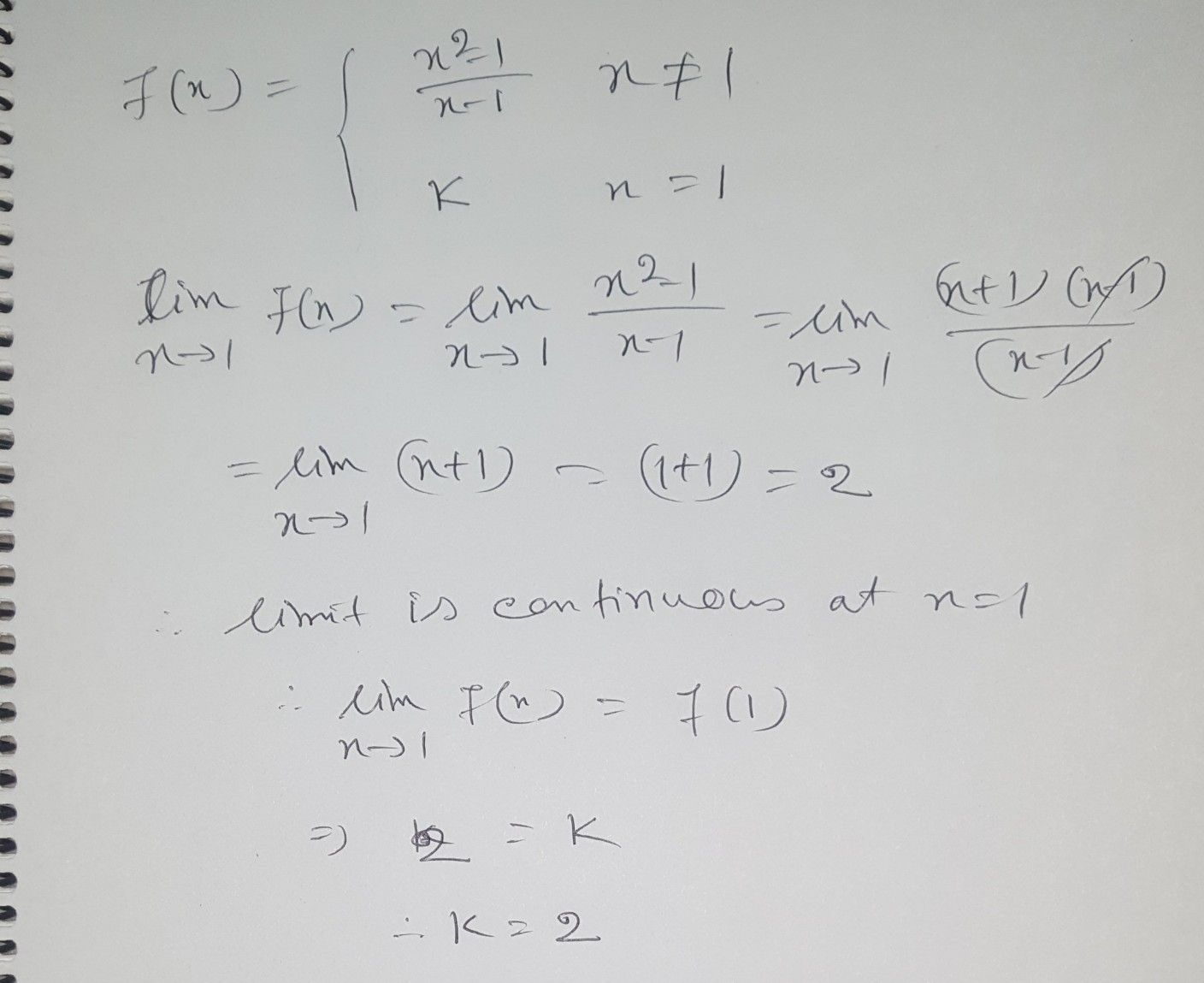Symbol
Problem$1$ $t=1,$ then 12. $2.H$ the function $f\left(x\right)= \begin{cases} \dfrac {x^{2}-1} {x-1}w \\ k \\ n \end{cases}$ when $x\left($ $1$ is given to be continuous at $x$ when $x=1$ $x+1$
10th-13th grade
Calculus
Search count: 170
SolutionQanda teacher - AnandiStudent
thank you! we appreciate your work! keep maintaining good quality of results:)
thank you! we appreciate your work! keep maintaining good quality of results:)